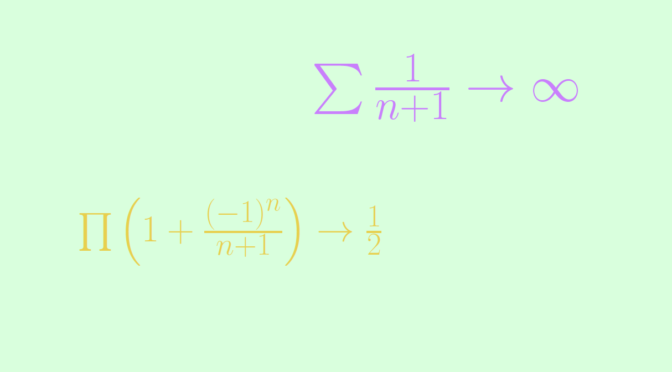# Counterexample around infinite products

Let’s recall two theorems about infinite products $$\prod \ (1+a_n)$$. The first one deals with nonnegative terms $$a_n$$.

THEOREM 1 An infinite product $$\prod \ (1+a_n)$$ with nonnegative terms $$a_n$$ converges if and only if the series $$\sum a_n$$ converges.

The second is related to infinite products with complex terms.

THEOREM 2 The absolute convergence of the series $$\sum a_n$$ implies the convergence of the infinite product $$\prod \ (1+a_n)$$. Moreover $$\prod \ (1+a_n)$$ is not zero providing $$a_n \neq -1$$ for all $$n \in \mathbb N$$.

The converse of Theorem 2 is not true as shown by following counterexample.

We consider $$a_n=(-1)^n/(n+1)$$. For $$N \in \mathbb N$$ we have:
$\prod_{n=1}^N \ (1+a_n) = \begin{cases} \frac{1}{2} &\text{ for } N \text{ odd}\\ \frac{1}{2}(1+\frac{1}{N+1}) &\text{ for } N \text{ even} \end{cases}$ hence the infinite product $$\prod \ (1+a_n)$$ converges (to $$\frac{1}{2}$$) while the series $$\sum \left\vert a_n \right\vert = \sum \frac{1}{n+1}$$ diverges (it is the harmonic series with first term omitted).